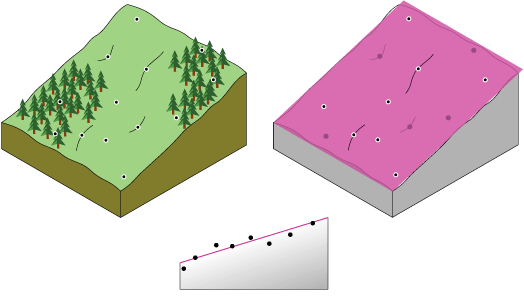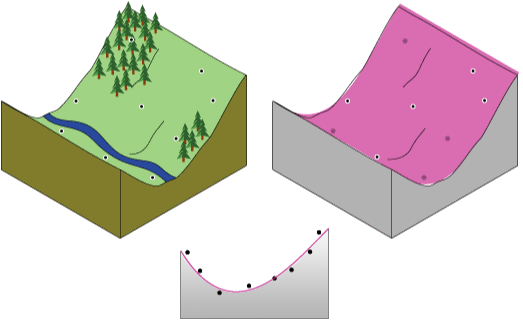# Subtracting out slope (in general) of DEM using ArcGIS for Desktop?

Subtract the trend surface from the DEM.

Linear trend (1st order polynomial)2nd order polynomial trendPer @radouxju's comment - the trend line can be shifted down to avoid negative values by adding the minimum value.

In the Raster Calculator:

"DEM" - Trend("DEM") + N


Where: N = Minimum raster value in the DEM

In python

import arcpy
from arcpy.sa import Trend, Raster
dem = 'c:/data/elevation.tif'
demmin = arcpy.GetRasterProperties_management(dem, "MINIMUM").getOutput(0)
dem = Raster(dem)
result = dem - Trend(dem) + demmin
result.save('c:/data/detrend.tif')